# Distance two imaginary numbs

Find the distance between two complex number: z1=(-8+i) and z2=(-1+i).

d =  7

### Step-by-step explanation: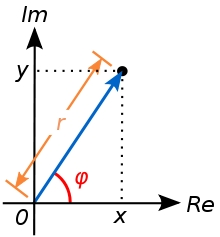Did you find an error or inaccuracy? Feel free to write us. Thank you!

Showing 1 comment:Math student
(16+13)(-20+23)Tips to related online calculators
Try our complex numbers calculator.
Do you want to convert length units?
Pythagorean theorem is the base for the right triangle calculator.

#### You need to know the following knowledge to solve this word math problem:

We encourage you to watch this tutorial video on this math problem:

## Related math problems and questions:

• Linear imaginary equationGiven that 2(z+i)=i(z+i) "this is z star" Find the value of the complex number z.
• ABS CNCalculate the absolute value of complex number -15-29i.
• Modulus and argument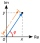Find the mod z and argument z if z=i
• Log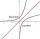Calculate value of expression log |3 +7i +5i2| .
• Goniometric form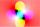Determine the goniometric form of a complex number z = √ 110 +4 i.
• The fenceI'm building a fence. Late is rounded up in semicircle. The tops of late in the field between the columns are to copy an imaginary circle. The tip of the first and last lath in the field is a circle whose radius is unknown. The length of the circle chord
• Imaginary numbersFind two imaginary numbers whose sum is a real number. How are the two imaginary numbers related? What is its sum?
• The modulus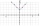Find the modulus of the complex number 2 + 5i
• Find the 5Find the equation of the circle with center at (1,20), which touches the line 8x+5y-19=0
• Distance problem 2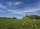A=(x,2x) B=(2x,1) Distance AB=√2, find value of x
• Space vectors 3DThe vectors u = (1; 3; -4), v = (0; 1; 1) are given. Find the size of these vectors, calculate the angle of the vectors, the distance between the vectors.
• Evaluate 18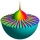Evaluate the expression (-4-7i)-(-6-9i) and write the result in the form a+bi (Real + i* Imaginary).
• Vertices of a right triangleShow that the points D(2,1), E(4,0), F(5,7) are vertices of a right triangle.
• IntegerFind the integer whose distance on the numerical axis from number 1 is two times smaller as the distance from number 6.
• Two chordsIn a circle with radius r = 26 cm two parallel chords are drawn. One chord has a length t1 = 48 cm and the second has a length t2 = 20 cm, with the center lying between them. Calculate the distance of two chords.
• Distance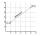Calculate distance between two points X[18; 19] and W[20; 3].
• Complex number coordinatesWhich coordinates show the location of -2+3i